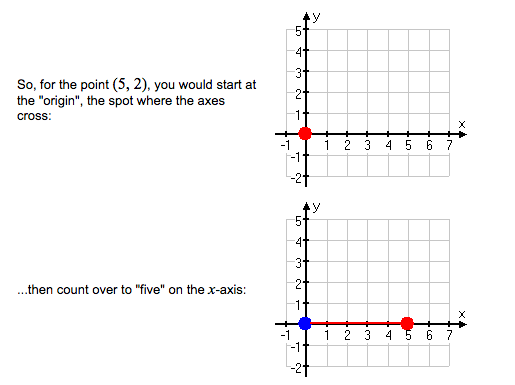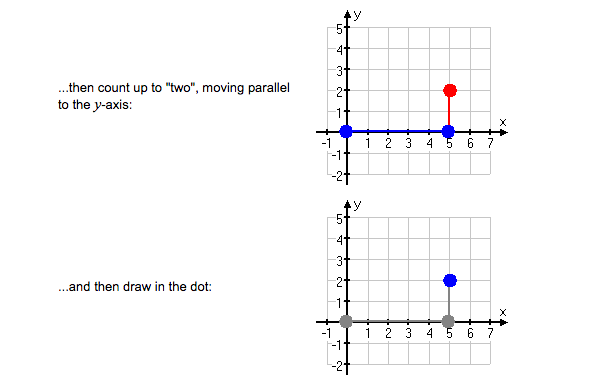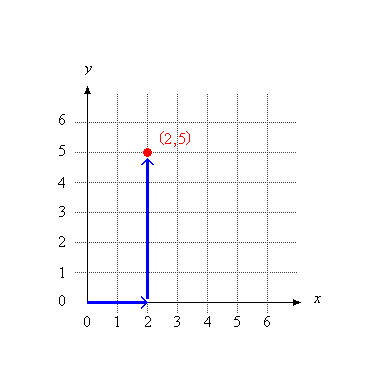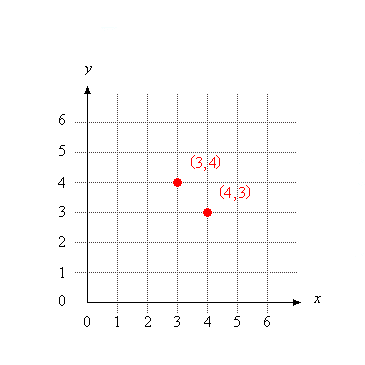Home MATHEMATICS TOPIC 10: COORDINATE OF A  POINT ~ MATHEMATICS FORM 1

# TOPIC 10: COORDINATE OF A  POINT ~ MATHEMATICS FORM 1

1036
1Read the coordinates of a point
Coordinates of a points – are the values of 𝑥 and 𝑦 enclosed by the bracket which are used to describe the position of a point in the plane
The plane used is called 𝑥𝑦 − plane and it has two axis; horizontal axis known as 𝑥 − axis and; vertical axis known as 𝑦 − axis
A Point Given its Coordinates
Plot a point given its coordinates
Suppose you were told to locate (5, 2) on the plane. Where would you look? To understand the meaning of (5, 2), you have to know the following rule:
Thex-coordinate (alwayscomes first. The first number (the first coordinate) isalwayson the horizontal axis.A Point on the Coordinates
Locate a point on the coordinates
The location of (2,5) is shown on the coordinate grid below. Thex-coordinate is 2. They-coordinate is 5. To locate (2,5), move 2 units to the right on thex-axis and 5 units up on they-axis.The order in which you writex– andy-coordinates in an ordered pair is very important. Th ex-coordinate always comes first, followed by they-coordinate. As you can see in the coordinate grid below, the ordered pairs (3,4) and (4,3) refer to two different points!#### 1 COMMENT

1.Fred mwanisawa

I fail to access other subject out of mathematics could assist me please